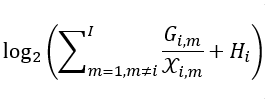How can I express the following formula in CVX？Where X is the variable，I tried many ways but it doesn’t work.

How to express log(1+ sum ( 1./x ) ) in CVX? and make the x_i be x_{i,m}/G_{i,m} and change the prepended 1 to 1/H_i

You can convert log2, which is not supported by CVX, to log by dividing by log(2).

1 Like